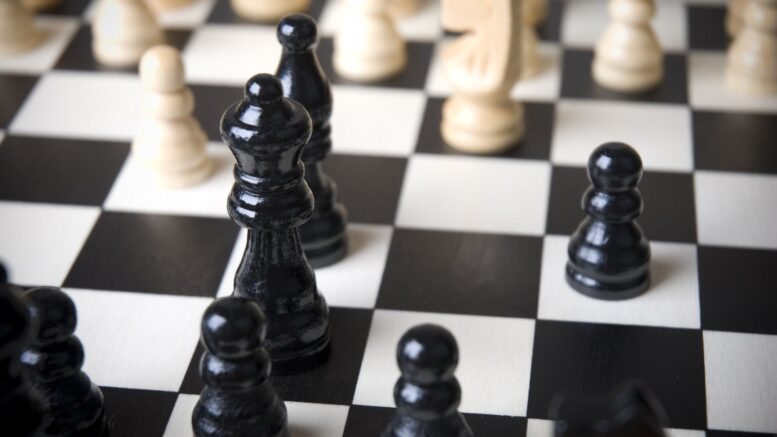# Mathematics On ChessboardBy Chandan Chakraborty

Mathematics is a very interesting subject. In our daily life, there is an enormous application of mathematics. Anyone without knowledge of basic withmatic may be cheated. So the students from the early-stage should learn to calculate withmatic, geometry, mensuration logically to avoid being cheated.

Chess is a mindgame, believed to be invented by the demon king Ravana to amuse his wife Mandodari, involves visualization, calculation with patience. To be a grandmaster (GM) in chess, a vigorous amount of toil and perseverance are highly important.

For a student reading at primacy level, chess can help increase imagination, patience, and ability to visualize. It has been observed during the Covid 19 situation that the students in tender age gradually becoming restless. For such students, Chess can be very helpful to concentrate and meditate.There are very interesting mathematical applications on chess boards that I have found in my research for years. These findings include multiplication, addition, factors, and multiples which are similar to arithmetic. At the same time, it has geometrical figures like squares, rectangles and has an area, perimeters, diagonals that are similar to mensuration.

These ideas are fit for primary-level students. However, for secondary and higher-level students, there are mathematical ideas like combinatorics, sum of squares, coloring problems, graph theory using the chessboard. All these are very helpful and joyful to develop basic ideas of mathematics among the students. Nevertheless, there is a bit of logo programming found in the movement of knights also.

Let us first see the arithmetic. On a chessboard, we can easily count tables of numbers from any side. For example, take 6 squares on one side and three squares on any adjustment side; we get a total of 6×3=18 squares in all. The students can either round all 18 squares or multiply 6×3 to get the same result! For factors and multipliers, we can take any 3×2=6 adjacent squares and check that these squares can be arranged also as 6×1=6 squares leading to the result that the numbers 6 have 4 factors 1, 2, 3, and 6 itself. So 6 is not a prime number.

In the case of geometry, students can easily find the squares and rectangles of different sizes and include different numbers of smallest squares. They can even draw diagonals and find their length to check Pythagoras theorem.  Each smallest square will have a length of the unit when each smallest side is assumed to be of 1 unit.

In case of mensuration, the area of quadrilaterals of size 2×2, 2×3, 4×7 or any such can be found and checked with the conventional formula length x breadth square unit. Perimeters can also be found by going over the outline borders of any combination of squares viz. 6 x 8 and calculating the number of smallest squares passed. For this example, the finger will pass through 6 + 8 + 6 + 8 = 2 (6+8) = 2×14=28 smallest squares. Hence the perimeter of 6×8 squares is 28 units. One unit is obviously the length of a side of the smallest square. Lastly, all the movement of the Knight ignites some logical thinking leading to logo programming turtle movement as follows –

FD 2 (Forward 2 steps)

RT 90 (Right turn 900)

FD 1

When a knight is in a central position it can jump to a maximum of eight possible squares all depending on a logical decision. So the students, knights of the future globe, are hereby advised to think logically, calculate physically and mentally with an ambition to know everything around them

Please play chess and learn most of it.

References:

Share the news